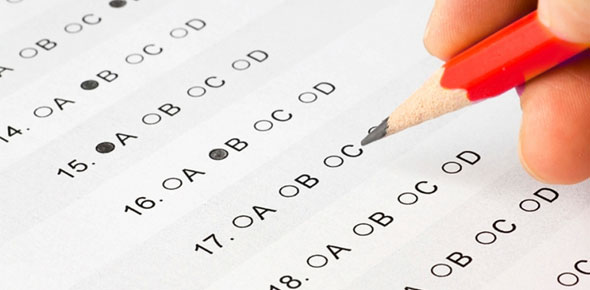# STAAR 7 Math Quiz#2

Approved & Edited by ProProfs Editorial Team
At ProProfs Quizzes, our dedicated in-house team of experts takes pride in their work. With a sharp eye for detail, they meticulously review each quiz. This ensures that every quiz, taken by over 100 million users, meets our standards of accuracy, clarity, and engagement.
| Written by Mathdancer
M
Mathdancer
Community Contributor
Quizzes Created: 3 | Total Attempts: 1,620
Questions: 10 | Attempts: 87SettingsOperations with Whole Numbers, Comparing & Ordering Decimals, and Multiply Decimals

• 1.

### Katie has a bag of 43 cookies. She and her 14 friends want cookies.  Does Katie have enough so that everyone can have 3 cookies?  Yes or No

Explanation
Since Katie has 43 cookies and there are 14 friends, the total number of people who want cookies is 14 + 1 (Katie) = 15. If each person wants 3 cookies, the total number of cookies needed would be 15 x 3 = 45. Since Katie only has 43 cookies, she does not have enough for everyone to have 3 cookies. Therefore, the answer is "No".

Rate this question:

• 2.

### Mrs. Glenn is making bookmarks with her students.  She wants to have 4 stickers for each student to use to make their bookmark.   If there are 17 students in her class, how many stickers should Mrs. Glenn buy?

Explanation
Mrs. Glenn wants to have 4 stickers for each student. Since there are 17 students in her class, she needs to buy 4 stickers for each student. Therefore, the total number of stickers she should buy is 17 multiplied by 4, which equals 68.

Rate this question:

• 3.

### Ella wants to buy pizza for her friends.  Each piece of pizza costs \$3.00.  Ella has \$18.00.  How many slices can she buy?

Explanation
Ella wants to buy pizza for her friends and each piece of pizza costs \$3.00. Ella has \$18.00. To find out how many slices she can buy, we divide the total amount of money she has by the cost of each slice. \$18.00 divided by \$3.00 equals 6. Therefore, Ella can buy 6 slices of pizza for her friends.

Rate this question:

• 4.

### What is the least decimal number between 1 and 1.5 that can be written using the digits 1,2,3,6,0

• A.

1.0632

• B.

1.2306

• C.

1.0362

• D.

1.0236

D. 1.0236
Explanation
The least decimal number between 1 and 1.5 that can be written using the digits 1, 2, 3, 6, 0 is 1.0236. This is because it is the smallest number that can be formed using these digits and falls within the given range.

Rate this question:

• 5.

### Which worker has the least hourly wage? Arlene \$15.50 Kathy \$18.50 Lucy \$18.75 Martha \$19.25

• A.

Arlene

• B.

Kathy

• C.

Lucy

• D.

Martha

A. Arlene
Explanation
Arlene has the least hourly wage because her wage is \$15.50, which is the lowest among all the given options.

Rate this question:

• 6.

### Philip has a wooden plank that is 4.2 m long.  He saws off a piece that is 2.44 m long.  What is the length of the other piece?

• A.

1.76 m

• B.

1.84 m

• C.

2.24 m

• D.

6.64

A. 1.76 m
Explanation
Philip has a wooden plank that is 4.2 m long. He saws off a piece that is 2.44 m long. To find the length of the other piece, we subtract the length of the sawed-off piece from the total length of the plank. Therefore, the length of the other piece is 4.2 m - 2.44 m = 1.76 m.

Rate this question:

• 7.

### Frances plans to drive her sister's home, which is 1400 miles away.  She drove 456.3 miles on Monday, 389.7 miles on Tuesday, and 318.6 miles Wednesday.  How much farther must she drive to reach her sister's home?

• A.

364.6 mi

• B.

264.6 mi

• C.

236.6 mi

• D.

235.4 mi

D. 235.4 mi
Explanation
Frances has already driven a total of 456.3 + 389.7 + 318.6 = 1164.6 miles. To reach her sister's home, which is 1400 miles away, she still needs to drive 1400 - 1164.6 = 235.4 miles. Therefore, the correct answer is 235.4 mi.

Rate this question:

• 8.

### How much will you be charged for buying 0.85 yard of fabric, if the fabric costs \$7.86?

• A.

\$7.71

• B.

\$7.01

• C.

\$6.68

• D.

\$0.67

C. \$6.68
Explanation
To find the cost of buying 0.85 yards of fabric, we need to multiply the cost per yard (\$7.86) by the number of yards (0.85). This can be calculated as 7.86 * 0.85 = \$6.678. Rounding this to two decimal places gives us \$6.68, which is the correct answer.

Rate this question:

• 9.

### Which of the following is true regarding  the product of 0.026 and 0.34?

• A.

The prodoct is greater than 1.

• B.

The product is greater than either factor.

• C.

The product is less than either factor.

• D.

The product has 4 decinal place.

C. The product is less than either factor.
Explanation
The product of 0.026 and 0.34 is less than either factor because both factors are less than 1. When multiplying two numbers less than 1, the product will always be smaller than either factor.

Rate this question:

• 10.

### Which is the sum of 8.89, 7.0986, and 0.32?

• A.

1.63086

• B.

16.3086

• C.

163.086

• D.

1630.86Back to top